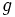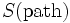# Least Action Principle

The Least Action Principle (LAP), or Principle of Least Action, was originally formulated by Pierre Louis Maupertuis, who stated that nature must always operate in the most efficient way possible, so all laws of nature should be described as the minimization of a certain quantity. Pierre Louis Maupertuis considered the Principle of Least Action as a proof that God exists.

In general terms, LAP dictates that:

• nature chooses the path of least distance and time, or the surface of least area
• nature expends the lowest deformation energy in deforming an elastic body

Adherence to the least-action principle enables identifying a unique solution from an infinite number of possibilities.

In classical mechanics, the Least Action Principle states that the motion of a particle along some path always minimizes the difference between its kinetic energy and its potential energy. Mathematically, the motion of a particle always minimizes the Lagrangian action functional.

In General Relativity, the Least Action Principle states that the motion of a particle on a surface M must be a geodesic of M, so it must minimize the geodesic functional. Additionally, David Hilbert originally discovered the field equations from applying the principle of least action to the action functional, where the minimization takes place over the space of metricson spacetime, anddenotes the scalar curvature of the metric.

In Quantum Mechanics, the amplitude for a particle start at point A and end at point B is given by the integral ofover the space of all paths joining A and B. Heredenotes the action of the path. Thus, by the stationary phase approximation, the amplitude receives the greatest contribution from the path that minimizes the action. This is the Feynman path integral approach to quantum mechanics.

Recently, mathematician Alain Connes has formulated a spectral action principle that seeks to apply the Least Action Principle to the mathematical formulation of quantum gravity.